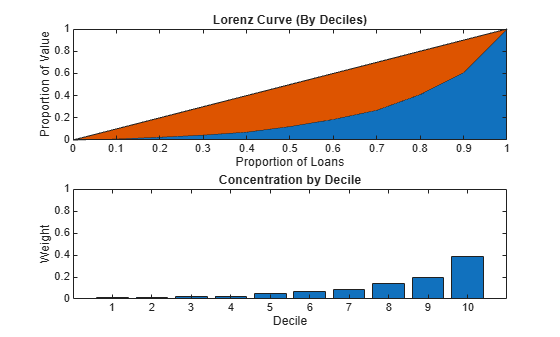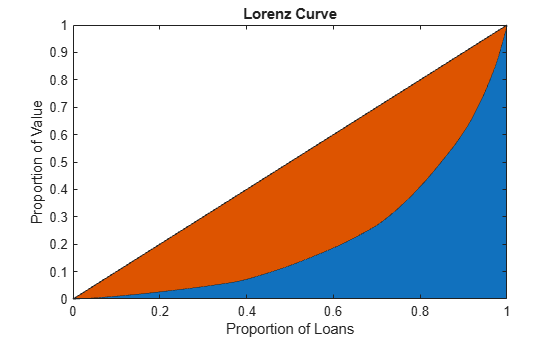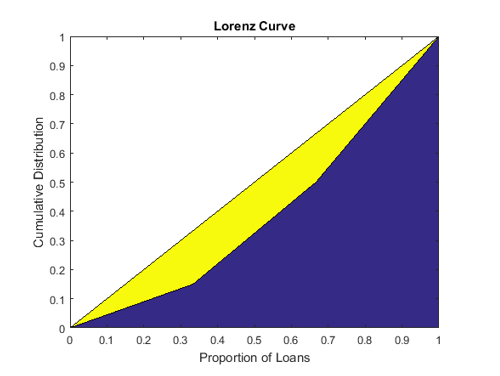# concentrationIndices

Compute ad-hoc concentration indices for a portfolio

## Syntax

``ci = concentrationIndices(PortfolioData)``
``````[ci,Lorenz] = concentrationIndices(___,Name,Value)``````

## Description

example

````ci = concentrationIndices(PortfolioData)` computes multiple ad-hoc concentration indices for a given portfolio. The `concentrationIndices` function supports the following indices: CR — Concentration ratioDeciles — Deciles of the portfolio weights distributionGini — Gini coefficientHH — Herfindahl-Hirschman indexHK — Hannah-Kay indexHT — Hall-Tideman indexTE — Theil entropy index ```

example

``````[ci,Lorenz] = concentrationIndices(___,Name,Value)``` adds optional name-value pair arguments. ```

## Examples

collapse all

Compute the concentration indices for a credit portfolio using a portfolio that is described by its exposures. The exposures at default are stored in the `EAD` array.

Load the `CreditPortfolioData.mat` file that contains `EAD` used for the `PortfolioData` input argument.

```load CreditPortfolioData.mat ci = concentrationIndices(EAD)```
```ci=1×8 table ID CR Deciles Gini HH HK HT TE ___________ ________ ___________ _______ ________ ________ ________ _______ "Portfolio" 0.058745 1x11 double 0.55751 0.023919 0.013363 0.022599 0.53485 ```

Use the `CRIndex` optional input to obtain the concentration ratios for the tenth and twentieth largest exposures. In the output, the `CR` column becomes a vector, with one value for each requested index.

Load the `CreditPortfolioData.mat` file that contains the `EAD` used for the `PortfolioData` input argument.

```load CreditPortfolioData.mat ci = concentrationIndices(EAD,'CRIndex',[10 20])```
```ci=1×8 table ID CR Deciles Gini HH HK HT TE ___________ __________________ ___________ _______ ________ ________ ________ _______ "Portfolio" 0.38942 0.58836 1x11 double 0.55751 0.023919 0.013363 0.022599 0.53485 ```

Use the `HKAlpha` optional input to set the alpha parameter for the Hannah-Kay (HK) index. Use a vector of alpha values to compute the `HK` index for multiple parameter values. In the output, the `HK` column becomes a vector, with one value for each requested alpha value.

Load the `CreditPortfolioData.mat` file that contains `EAD` used for the `PortfolioData` input argument.

```load CreditPortfolioData.mat ci = concentrationIndices(EAD,'HKAlpha',[0.5 3])```
```ci=1×8 table ID CR Deciles Gini HH HK HT TE ___________ ________ ___________ _______ ________ ____________________ ________ _______ "Portfolio" 0.058745 1x11 double 0.55751 0.023919 0.013363 0.029344 0.022599 0.53485 ```

Compare the concentration measures using an `ID` optional argument for a fully diversified portfolio and a fully concentrated portfolio.

```ciD = concentrationIndices([1 1 1 1 1],'ID','Fully diversified'); ciC = concentrationIndices([0 0 0 0 5],'ID','Fully concentrated'); disp([ciD;ciC])```
``` ID CR Deciles Gini HH HK HT TE ____________________ ___ ___________ ____ ___ ___ ___ ___________ "Fully diversified" 0.2 1x11 double 0 0.2 0.2 0.2 -2.2204e-16 "Fully concentrated" 1 1x11 double 0.8 1 1 1 1.6094 ```

Use the `ScaleIndices` optional input to scale the index values of `Gini`, `HH`, `HK`, `HT`, and `TE`. The range of `ScaleIndices` is from `0` through `1`, independent of the number of loans.

```ciDU = concentrationIndices([1 1 1 1 1],'ID','Diversified, unscaled'); ciDS = concentrationIndices([1 1 1 1 1],'ID','Diversified, scaled','ScaleIndices',true); ciCU = concentrationIndices([0 0 0 0 5],'ID','Concentrated, unscaled'); ciCS = concentrationIndices([0 0 0 0 5],'ID','Concentrated, scaled','ScaleIndices',true); disp([ciDU;ciDS;ciCU;ciCS])```
``` ID CR Deciles Gini HH HK HT TE ________________________ ___ ___________ ____ __________ ___________ ___________ ___________ "Diversified, unscaled" 0.2 1x11 double 0 0.2 0.2 0.2 -2.2204e-16 "Diversified, scaled" 0.2 1x11 double 0 3.4694e-17 -3.4694e-17 -6.9389e-17 -1.3796e-16 "Concentrated, unscaled" 1 1x11 double 0.8 1 1 1 1.6094 "Concentrated, scaled" 1 1x11 double 1 1 1 1 1 ```

Load the `CreditPortfolioData.mat` file that contains EAD used for the `PortfolioData` input argument.

```load CreditPortfolioData.mat P = EAD; ci = concentrationIndices(P);```

Visualize an approximate Lorenz curve using the deciles information and also the concentration at the decile level.

```Proportion = 0:0.1:1; figure; subplot(2,1,1) area(Proportion',[ci.Deciles' Proportion'-ci.Deciles']) axis([0 1 0 1]) title('Lorenz Curve (By Deciles)') xlabel('Proportion of Loans') ylabel('Proportion of Value') subplot(2,1,2) bar(diff(ci.Deciles)) axis([0 11 0 1]) title('Concentration by Decile') xlabel('Decile') ylabel('Weight')```Load the `CreditPortfolioData.mat` file that contains the `EAD` used for the `PortfolioData` input argument. The optional output `Lorenz` contains the data for the exact Lorenz curve.

```load CreditPortfolioData.mat P = EAD; [~,Lorenz] = concentrationIndices(P); figure; area(Lorenz.ProportionLoans,[Lorenz.ProportionValue Lorenz.ProportionLoans-Lorenz.ProportionValue]) axis([0 1 0 1]) title('Lorenz Curve') xlabel('Proportion of Loans') ylabel('Proportion of Value')```## Input Arguments

collapse all

Nonnegative portfolio positions in N assets, specified as an `N`-by-`1` (or `1`-by-`N`) numeric array.

Data Types: `double`

### Name-Value Arguments

Specify optional comma-separated pairs of `Name,Value` arguments. `Name` is the argument name and `Value` is the corresponding value. `Name` must appear inside quotes. You can specify several name and value pair arguments in any order as `Name1,Value1,...,NameN,ValueN`.

Example: `[ci,Lorenz] = concentrationIndices(PortfolioData,'CRIndex',100)`

Index of interest for the concentration ratio, specified as the comma-separated pair consisting of `'CRIndex'` and an integer value between `1` and N, where N is the number of assets in the portfolio. The default value for `CRIndex` is `1` (the default `CR` is the largest portfolio weight). If `CRIndex` is a vector, the concentration ratio is computed for the index value in the given order.

Data Types: `double`

Alpha parameter for Hannah-Kay index, specified as the comma-separated pair consisting of `'HKAlpha'`, and a positive number that cannot be equal to `1`. If `HKAlpha` is a vector, the Hannah-Kay index is computed for each alpha value in the given order.

Data Types: `double`

User-defined ID for the portfolio, specified as the comma-separated pair consisting of `'ID'` and a scalar string object or character vector.

Data Types: `char` | `string`

Flag to indicate whether to scale concentration indices, specified as the comma-separated pair consisting of `'ScaleIndices'` and a logical scalar. When the `ScaleIndices` is set to true, the value of the `Gini`, `HH`, `HK`, `HT`, and `TE` indices are scaled so that all these indices have a minimum value of `0` (full diversification) and a maximum value of `1` (full concentration).

Note

Scaling is applied only for portfolios with at least two assets. Otherwise, the scaling capability is undefined.

Data Types: `logical`

## Output Arguments

collapse all

Concentration indices information for the given portfolio, returned as a table with the following columns:

• `ID` — Portfolio ID string. Use the `ID` name-value pair argument to set it.

• `CR` — Concentration ratio. By default, the concentration ratio for the first index (largest portfolio weight) is reported. Use the `CRIndex` name-value pair argument to choose a different index. If `CRIndex` is a vector of length m, then `CR` is a row vector of size `1`-by-m. For more information, see More About.

• `Deciles` — Deciles of the portfolio weights distribution is a `1`-by-`11` row vector containing the values `0`, the nine decile cut points, and `1`. For more information, see More About.

• `Gini` — Gini coefficient. For more information, see More About.

• `HH` — Herfindahl-Hirschman index. For more information, see More About.

• `HK` — Hannah-Kay index (reciprocal). By default, the `'alpha'` parameter is set to `0.5`. Use the `HKAlpha` name-value pair argument to choose a different value. If `HKAlpha` is a vector of lengthm, then `HK` is a row vector of size `1`-by-m. For more information, see More About.

• `HT` — Hall-Tideman index. For more information, see More About.

• `TE` — Theil entropy index. For more information, see More About.

Lorenz curve data, returned as a table with the following columns:

• `ProportionLoans` — (`N`+`1`)-by-`1` numeric array containing the values `0`, `1`/`N`, `2`/`N`, ... `N`/`N` = `1`. This is the data for the horizontal axis of the Lorenz curve.

• `ProportionValue` — (`N`+`1`)-by-`1` numeric array containing the proportion of portfolio value accumulated up to the corresponding proportion of loans in the `ProportionLoans` column. This is the data for the vertical axis of the Lorenz curve.

collapse all

### Portfolio Notation

All the concentration indices for `concentrationIndices` assume a credit portfolio with an exposure to counterparties.

Let P be a given credit portfolio with exposure to N counterparties. Let x1,...xN represent the exposures to each counterparty, with xi > = `0` for all i = `1`,...N. And, let x be the total portfolio exposure

`$x=\sum _{i=1}^{N}{x}_{i}$`

Assume that x > `0`, that is, at least one exposure is nonzero. The portfolio weights are given by w1,...,wN with

`${w}_{i}=\frac{{x}_{i}}{x}$`

The weights are sorted in non-decreasing order. The following standard notation uses brackets around the indices to denote ordered values.

`${w}_{\left[1\right]}\le {w}_{\left[2\right]}\le ...\le {w}_{\left[N\right]}$`

### Concentration Ratio

The concentration ratio (CR) answers the question “what proportion of the total exposure is accumulated in the largest k loans?”

The formula for the concentration ratio (CR) is:

`$C{R}_{k}=\sum _{i=1}^{k}{w}_{{}_{\left[N-i+1\right]}}$`

For example, if k=`1`, CR1 is a sum of the one term w[N-1+1] = w[N], that is, it is the largest weight. For any k, the CR index takes values from `0` through `1`.

### Lorenz Curve

The Lorenz curve is a visualization of the cumulative proportion of portfolio value (or cumulative portfolio weights) against the cumulative proportion of loans.

The cumulative proportion of loans (p) is defined by:

`${p}_{0}=0,{p}_{1}=\frac{1}{N},{p}_{2}=\frac{2}{N},...,{p}_{N}=\frac{N}{N}=1$`

The cumulative proportion of portfolio value L is defined as:

`${L}_{0}=0,{L}_{k}={\sum }_{i=1}^{k}{w}_{\left[i\right]}$`

The Lorenz curve is a plot of L versus p, or the cumulative proportion of portfolio value versus cumulative proportion of the number of loans (sorted from smallest to largest).The diagonal line is indicated in the same plot because it represents the curve for the portfolio with the least possible concentration (all loans with the same weight). The area between the diagonal and the Lorenz curve is a visual representation of the Gini coefficient, which is another concentration measure.

### Deciles

Deciles are commonly used in the context of income inequality.

If you sort individuals by their income level, what proportion of the total income is earned by the lowest 10% and the lowest 20% of the population? In a credit portfolio, loans can be sorted by exposure. The first decile corresponds to the proportion of the portfolio value that is accumulated by the smallest 10% loans, and so on. Deciles are proportions, therefore they always take values from `0` through `1`.

Defining the cumulative proportion of loans (p) and the cumulative proportion of values L as in Lorenz Curve, the deciles are a subset of the proportion of value array. Given indices d1, d2,…, d9 such that the proportion of loans matches exactly these values:

`${p}_{d1}=0.1,{p}_{d2}=0.2,...,{p}_{d9}=0.9$`

The deciles D0,D1,....,D9,D10 are defined as the corresponding proportion of values:

`${D}_{0}={L}_{0}=0,{D}_{1}={L}_{d1},{D}_{2}={L}_{d2},...,{D}_{9}={L}_{d9,}{D}_{10}={L}_{N}=1$`

When the total number of loans N is not divisible by 10, no indices match the exact proportion of loans 0.1, 0.2, and so on. In that case, the decile values are linearly interpolated from the Lorenz curve data (that is, from the p and L arrays). With this definition, there are 11 values in the deciles information because the end points 0% and 100% are included.

### Gini Index

The Gini index (or coefficient) is visualized on a Lorenz curve plot as the area between the diagonal and the Lorenz curve.

Technically, the Gini index is the ratio of that area to the area of the full triangle under the diagonal on the Lorenz curve plot. The Gini index is also defined equivalently as the average absolute difference between all the weights in the portfolio normalized by the average weight.

Using the proportion of values that array L defined in the Lorenz curve section, the Gini index is given by the formula:

`$Gini=1-\frac{1}{N}{\sum }_{i=1}^{N}\left({L}_{i-1}+{L}_{i}\right)$`

Equivalently, the Gini index can be computed from the sorted weights directly with the formula:

`$Gini=\frac{1}{N}{\sum }_{i=1}^{N}\left(2i-1\right){w}_{\left[i\right]}-1$`

The Gini coefficient values are always between `0` (full diversification) and `1``1`/N (full concentration).

### Herfindahl-Hirschman Index

The Herfindahl-Hirschman index is commonly used as a measure of market concentration.

The formula for the Herfindahl-Hirschman index is:

`$HH={\sum }_{i=1}^{N}{w}_{i}{}^{2}$`

The Herfindahl-Hirschman index takes values between `1`/N (full diversification) and `1` (full concentration).

### Hannah-Kay Index

The Hannah-Kay index is a generalization of the Herfindahl-Hirschman index.

The formula for the Hannah-Kay depends on a parameter α > `0`, α ≠ `1`, as follows:

`$H{K}_{\alpha }={\left({\sum }_{i=1}^{N}{w}_{i}{}^{\alpha }\right)}^{1/\left(\alpha -1\right)}$`

This formula is the reciprocal of the original Hannah-Kay index, which is defined with `1`/(`1`− α) in the exponent. For concentration analysis, the reciprocal formula is the standard because it increases as the concentration increases. This is the formula implemented in `concentrationIndices`. The Hannah-Kay index takes values between `1`/N (full diversification) and `1` (full concentration).

### Hall-Tideman Index

The Hall-Tideman index is a measure commonly used for market concentration.

The formula for the Hall-Tideman index is:

`$HT=\frac{1}{2{\sum }_{i=1}^{N}\left(N-i+1\right){w}_{\left[i\right]}-1}$`

The Hall-Tideman index takes values between `1`/N (full diversification) and `1` (full concentration).

### Theil Entropy Index

The Theil entropy index, based on a traditional entropy measure (for example, Shannon entropy), is adjusted so that it increases as concentration increases (entropy moves in the opposite direction), and shifted to make it positive.

The formula for the Theil entropy index is:

`$TE={\sum }_{i=1}^{N}{w}_{i}\mathrm{log}\left({w}_{i}\right)+\mathrm{log}\left(N\right)$`

The Theil entropy index takes values between `0` (full diversification) and `log`(N) (full concentration).

 Basel Committee on Banking Supervision. "Studies on Credit Risk Concentration". Working paper no. 15. November, 2006.

 Calabrese, R., and F. Porro. "Single-name concentration risk in credit portfolios: a comparison of concentration indices." working paper 201214, Geary Institute, University College, Dublin, May, 2012.

 Lütkebohmert, E. Concentration Risk in Credit Portfolios. Springer, 2009.